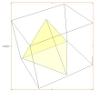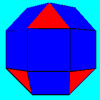#### You may also like### A Mean Tetrahedron

Can you number the vertices, edges and faces of a tetrahedron so that the number on each edge is the mean of the numbers on the adjacent vertices and the mean of the numbers on the adjacent faces?### Rhombicubocts

Each of these solids is made up with 3 squares and a triangle around each vertex. Each has a total of 18 square faces and 8 faces that are equilateral triangles. How many faces, edges and vertices does each solid have?### Icosian Game

This problem is about investigating whether it is possible to start at one vertex of a platonic solid and visit every other vertex once only returning to the vertex you started at.

# Which Solids Can We Make?

##### Age 11 to 14Challenge Level

Thank you to Ella from Trinity C of E School for sending us Euclid's geometric proof that there are no more platonic solids.

Each vertex of any solid must coincide with more than two faces. At each vertex of the solid the angle must be less than $360^{\circ}$. Since the angles at all the vertices of all the faces of a Platonic solid are identical, each vertex of each face must be less than ${360^{\circ}\over{3}}=120^{\circ}$. Only regular polygons with less than 6 sides have angles less than $120^{\circ}$ so the common face must be a triangle, square or pentagon and only the listed solids can be made with these faces.

Camilla from St Bernard's School sent us her workings for the angle deficit.

Tetrahedron: 3 triangles. $60^{\circ}\times3=180^{\circ}$ and $360^{\circ}-180^{\circ}=180^{\circ}$

Cube: 3 squares. $90^{\circ}\times3=270^{\circ}$ and $360^{\circ}-270^{\circ}=90^{\circ}$

Octahedron: 4 triangles. $60^{\circ}\times4=240^{\circ}$ and $360^{\circ}-240^{\circ}=120^{\circ}$

Icosahedron: 5 triangles. $60^{\circ}\times5=300^{\circ}$ and $360^{\circ}-300^{\circ}=60^{\circ}$

She also worked out that the total angle deficit is no. of vertices $\times$ angle deficit. For Platonic solids this is always $720^{\circ}$. Here is her working for the Archimedean solid.

{3, 3, 3, 3, 4} is 4 triangles and 1 square. $60^{\circ}\times4+90^{\circ}\times1=330^{\circ}$ and $360^{\circ}-330^{\circ}=30^{\circ}$. Number of vertices is 24 so the total angle deficit is $30^{\circ}\times24=720^{\circ}$ which is the same as a Platonic solid.

You can use this method to test whether vertex forms will work. For example, {4, 5, 8} is 1 square, 1 pentagon and 1 octagon. $90^{\circ}+108^{\circ}+135^{\circ}=333^{\circ}$ and $360^{\circ}-333^{\circ}=27^{\circ}$ and ${720^{\circ}\over27^{\circ}}=26.7$ but you can't have a non-integer number of vertices so this doesn't work. Whereas, {3, 8, 8} is 1 triangle and 2 octagons. $60^{\circ}\times1+135^{\circ}\times2=330^{\circ}$ and $360^{\circ}-330^{\circ}=30^{\circ}$ and ${720^{\circ}\over30^{\circ}}=24$ which does work.